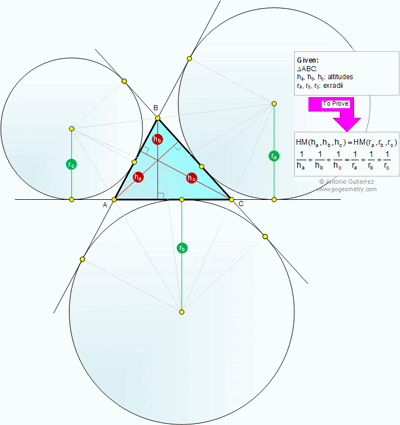## Friday, June 15, 2012

Geometry Problem
Level: Mathematics Education, High School, Honors Geometry, College.

Click the figure below to see the complete problem 766.#### 1 comment:

1.Jacob Ha & W FungJune 16, 2012 at 3:28 AM

Let Δ be area of triangle ABC, s = (a+b+c)/2

Hence,
1/(Ra) + 1/(Rb) = (s-a)/Δ + (s-b)/Δ =c/Δ = 2/(Hc)

Symmetrically,
1/(Ra) + 1/(Rb) =2/(Hc)
1/(Rb) + 1/(Rc) =2/(Ha)
1/(Rc) + 1/(Ra) =2/(Hb)

Summing up,

1/(Ra) + 1/(Rb) + 1/(Rc) = 1/(Ha) + 1/(Hb) + 1/(Hc)

q.e.d